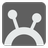Hubspot Amulet
Simple+
English

While exploring the island, Sofia found an interesting Amulet. Along the perimeter of the amulet small points are marked on each degree (for a total 360 degrees.) Three levers extrude from the amulet with varying lengths. The first lever is the shortest, the second lever is in the middle of the three and the last is the longest. These levers can be rotated, but if you rotate one lever the others rotate as well. The levers rotate discretely by degrees, rotating them clockwise gives you a positive angle while counterclockwise is negative.

After some experiments Sophie discovers the levers correlation. If you rotate a lever,...
If you rotate the first, then the second is rotated times faster, the third -- at the f3time faster.
If you rotate the second, then the third is rotated s3 times faster, the first -- at the s1 time faster.
If you rotate the third, then the first is rotated t1 times faster, the second -- at the t2 time faster.
This correlation can be represented as a matrix 3x3 with 1 in the main diagonal.
 1 f2 f3 s1 1 s3 t1 t2 1
For example, the rotating correlation is given as the matrix:
 1 2 3 3 1 2 2 3 1
This means that:
If you rotate the first, the second is rotated at double the angle while the third rotates at triple.
If you rotate the second, the third is rotated at double the angle, and the first rotates at triple.
If you rotate the third, the first is rotated at double the angle, and the second rotates at triple.
For example: You rotate the first lever at +10 degrees. As a result, the second is rotated at +20 degrees and the third at +30 degrees.

You can rotate the levers from -180 to +180 degrees only (but in interrelated mode it can be rotated more than 360 degrees). You can rotate each lever once in the following order: the first, the second, the third. Sophie used her X-ray vision and found out what position each lever should be in. After completing these rotations, the levers must be at 0, 225, 315.

You are given a rotation matrix as a list of lists with positive integers and the unitary main diagonal. You should return a list of three integers -- the angles for each lever. The start position of the levers is [0, 0, 0].Input: A rotation matrix as a list of lists.

Output: The angles as a list of integers.

Example:

```checkio([
[1, 2, 3],
[3, 1, 2],
[2, 3, 1]])
checkio([
[1, 4, 2],
[2, 1, 2],
[2, 2, 1]])
```

How it is used: This a funny combinatorial problem that could help you on dangerous journey in remote jungle for an ancient treasure, as we all know, ancient civilizations hide their treasure with tricky puzzles! ;-)

Precondition: ∀ x ∈ matrix : 0 < x < 10
|matrix| = 3x3

You should be an authorized user in order to see the full description and start solving this mission.